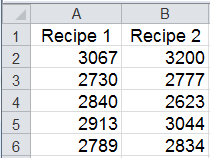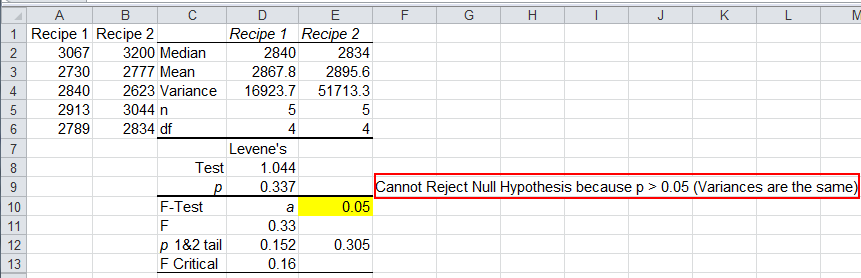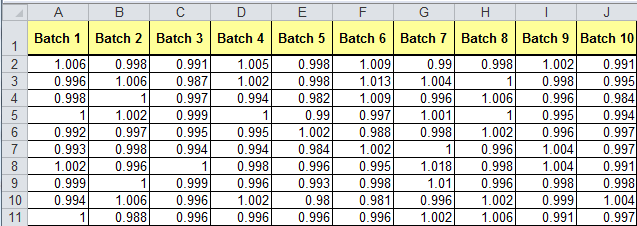# Levene's Test in Excel using QI Macros Add-in

## Levene's Test for Non-normal Data and More Than Two Data Samples

Levene's Test compares two or more independent sets of test data. It helps determine if the variances are the same or different from each other. The Levene's Test is like the f test. However, the Levene's test is robust enough for non-normal data and handles more than two columns of data. QI Macros uses Median (Brown-Forsythe modification) instead of the Mean for calculations.

Note: Excel does not do a Levene's Test; QI Macros adds this functionality.

### Levene's Test Example

If you're producing rubber made with two different recipes, you might want to know if the variances in tensile strengths are the same or different (Juran's QC Handbook 4th pg 23.74):

Conduct a test and enter the data into Excel.Click and drag over the data to select it and click on QI Macros Menu, Statistical Tools, f and t Tests and then Levene's test for variance:QI Macros will perform the calculations and interpret the results for you.### Interpreting the Levene's Test Results Manually

• The null hypothesis (H0) - variances are the same (variances are not different)
• The alternate hypothesis (Ha) - variances are different
 Hypothesis Test Compare Result p value Method p value < a Reject the null hypothesis p value Method p value > a Cannot Reject the null hypothesis (Accept the null hypothesis)

Since Levene's p value > a ( 0.337> 0.05), we cannot reject the null hypothesis (accept the null hypothesis) that the variances are equal.

While an F test works well on two-samples of normal data, it isn't robust enough to handle non-normal data or more than two samples. (Notice that Levene's p value differs from F Test's two-tailed value of 0.305; however both cause acceptance of null hypothesis.)

### Levene's Test - ten sample example

Now, consider the following example of ten batches of gear diameters:### Affordable

• Only \$329 USD - less with quantity discounts
• No annual fees
• Free Technical Support### Easy to Use

• Works Right in Excel
• Interprets Results for You
• Accurate Results Without Worry### Proven and Trusted

• 100,000 Users in 80 Countries
• Celebrating 20th Anniversary
• Five Star CNET Rating - Virus Free